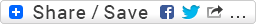## Python pitfall: Passing Mutable Objects as Default Args

I have a long and nested lists of parameters to throw out with a django template, but the visualization routine in the template processes them better if the parameters are ordered two by two. So I was trying to put together a simple function for reordering the original list accordingly. This is what I came up with:

```def group_list_items_by_two(lista, listaexit= []):
lista_x = []
if lista:
lista_x.extend(lista)
lista_x.reverse()

first_el = lista_x.pop()
if len(lista_x) == 0:
second_el = None
else:
second_el = lista_x.pop()
listaexit.append([first_el, second_el])
if lista[2:]:
group_list_items_by_two(lista[2:], listaexit)
#print(listaexit)
return listaexit
```

Nothing too extraordinary till here -I’m quite sure I could have done this in a better way (been wondering if there is a way to iterate over a list two elements at a time..but hey.. still learning python!) – however the function had a weird behaviour:

```>>> x = [('aaaa', 6), ('bbbb', 7), ('cccc', 8)]
>>> group_list_items_by_two(x)
[[('aaaa', 6), ('bbbb', 7)], [('cccc', 8), None]]
>>> group_list_items_by_two(x)
[[('aaaa', 6), ('bbbb', 7)], [('cccc', 8), None], [('aaaa', 6), ('bbbb', 7)],
... [('cccc', 8), None]]
>>> group_list_items_by_two(x)
[[('aaaa', 6), ('bbbb', 7)], [('cccc', 8), None], [('aaaa', 6), ('bbbb', 7)],
....[('cccc', 8), None], [('aaaa', 6), ('bbbb', 7)], [('cccc', 8), None]]
>>> x
[('aaaa', 6), ('bbbb', 7), ('cccc', 8)]
```

Basically – python is not using the default argument I’m passing (listaexit= []) and instead it keeps adding to the same variable. WHY IS this totally counter-intuitive behaviour happening?

A little bit of googling gave me an answer…

When you use a mutable object as a default arg, Python creates a single object, and reuses that same object on every call. I find this non-intuitive, personally, but that’s the way Python works.

The reason for this behaviour is that expressions in default arguments are calculated when the function is defined, not when it’s called (read this as well).
The solution is to pass None as the default value, and then add a line ‘listaexit = listaexit or []’ to avoid None type floating around….

And here’s a working version of the function above:

```def group_list_items_by_two(lista, listaexit= None):
lista_x = []
listaexit = listaexit or []
if lista:
lista_x.extend(lista)
lista_x.reverse()

first_el = lista_x.pop()
if len(lista_x) == 0:
second_el = None
else:
second_el = lista_x.pop()
listaexit.append([first_el, second_el])
if lista[2:]:
group_list_items_by_two(lista[2:], listaexit)
#print(listaexit)
return listaexit
```